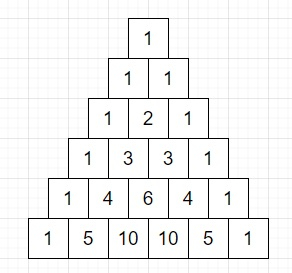# Program to generate Pascal's triangle in Python

Suppose we have a number n. We have to generate Pascal's triangle up to n lines. The Pascal's triangle will be look like this −The property of Pascal's triangle is the sum of each adjacent two numbers of previous row is the value of the number which is placed just below on the second row. For example, the first 10 at row 6 is sum of 4 and 6 at row 5 and second 10 is sum of two numbers 6 and 4 at row 5.

So, if the input is like n = 5, then the output will be

    1
1 1
1 2 1
1 3 3 1
1 4 6 4 1

To solve this, we will follow these steps −

• for i in range 0 to n+1, do
• for j in range 0 to n-i, do
• print one blank space
• C := 1
• for j in range 1 to i+1, do
• print C then a single blank space
• C := quotient of (C *(i - j) / j)
• go to next line

## Example

Let us see the following implementation to get better understanding −

def solve(n):
for i in range(n+1):
for j in range(n-i):
print(' ', end='')

C = 1
for j in range(1, i+1):
print(C, ' ', sep='', end='')
C = C * (i - j) // j
print()

n = 5
solve(n)

## Input

5

## Output

    1
1 1
1 2 1
1 3 3 1
1 4 6 4 1